back to all posts

# JavaScript Crash Course for Beginners -3

#### by Nabendu Biswas / August 27th, 2020

##### Series: JS-Basics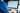Welcome to part-3 of the series. We will start with loops in this part.

### Loops

The first loop which will look is the traditional for loop, which is their in all languages.

Let’s look into the for loop below -

``````    for(let i=0; i <= 10; i++){
console.log(`Loop Number - \${i}`);
}
``````

Now, the flow of the loop is like for the first time let i=0 will run. After that i <= 10 will run. Now it will be true because 0 is less than or equal to 10. And, so the output of Loop Number- 0 will be printed.

Next, time the i++ will run, which is equivalent to i = i + 1. So, the i is now 1. Again the condition i <= 10 will run. Now it will be true because 1 is less than or equal to 10. And, so the output of Loop Number- 1 will be printed.

The loop will run till i is 10 and will print the outputs. Now, when i will be 11 after the increment and the condition i <= 10 will run. It will be false because 10 is less then equal to 10 will be false. So, the loop will end and come out.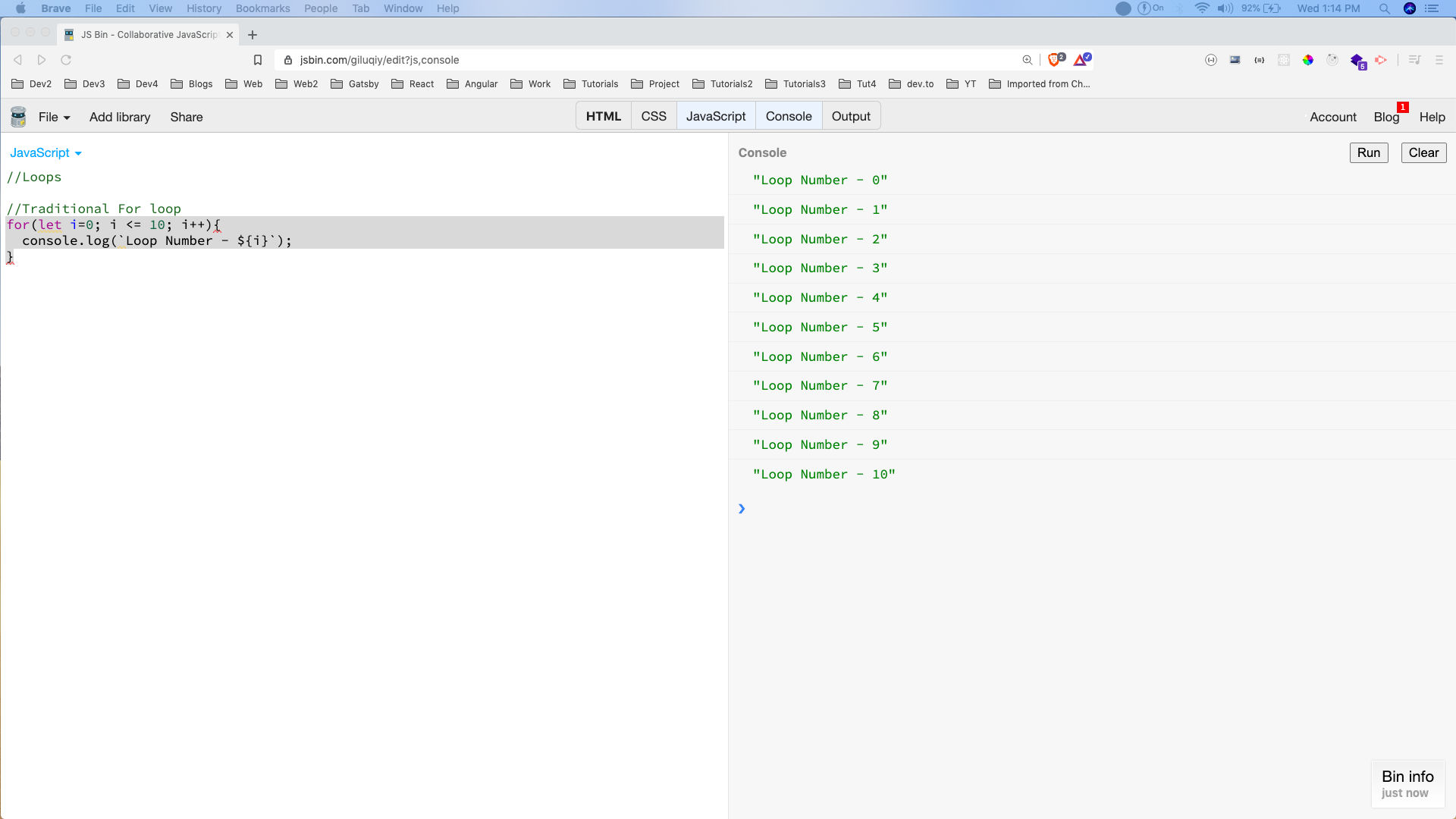For loop

We will look into one more traditional loop now and it is called the while loop. We will convert the above for loop into a while loop.

``````    let i = 0;
while(i <= 10) {
console.log(`Loop Number - \${i}`);
i++;
}
``````

It will run the initialisation first which is let i=0. After that it will enter the loop and check whether i <= 10. Now it will be true because 0 is less than or equal to 10. And, so the output of Loop Number- 0 will be printed.

Notice that we are incrementing i inside the loop only. So, i will now become 1 and the loop will be run again and the output of Loop Number- 1 will be printed.

The loop will run till i is 10 and will print the outputs. Now, when i will be 11 after the increment and the condition i <= 10 will run. It will be false because 10 is less than equal to 10 will be false. So, the loop will end and come out.

One important thing to keep in mind is to not forget to put the i++ inside the loop or else it will turn to an infinite loop.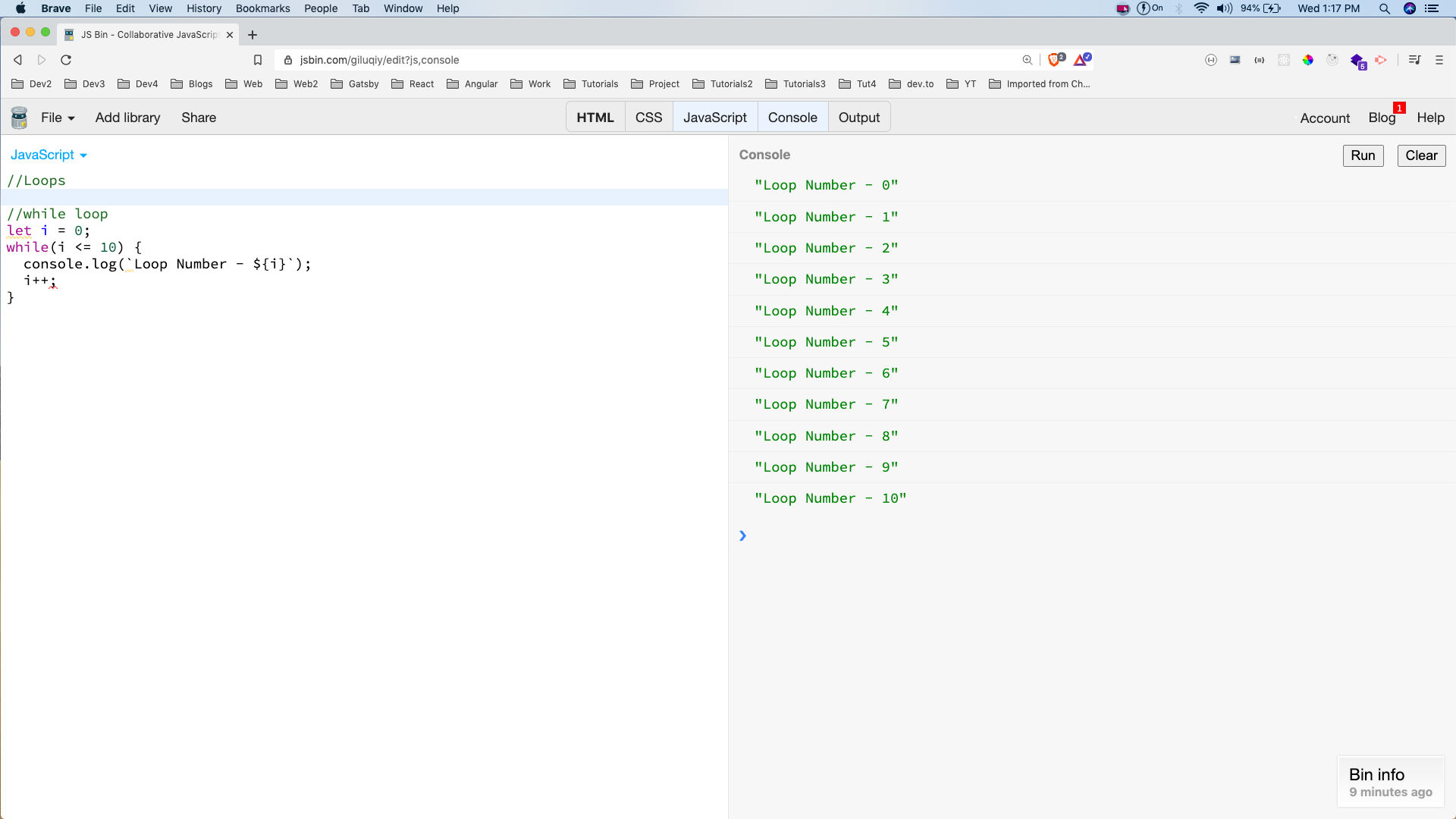while loop

Next, we will look into a variance of while loop called the do while loop. If you run the below loop, it will run exactly as the for or while loop.

``````    let i = 0;
do {
console.log(`Loop Number - \${i}`);
i++;
} while(i <= 10);
``````

But the main difference to understand is that it is not checking any condition at the start of the loop. So, even if the condition is not true it will run for one time. The below loop only runs once as the condition num < 5 is false after the first run.

``````    let num = 0;
do{
console.log(`Do while single - \${num}`);
num+=5;
}while(num < 5);
``````

Both of the outputs are shown below.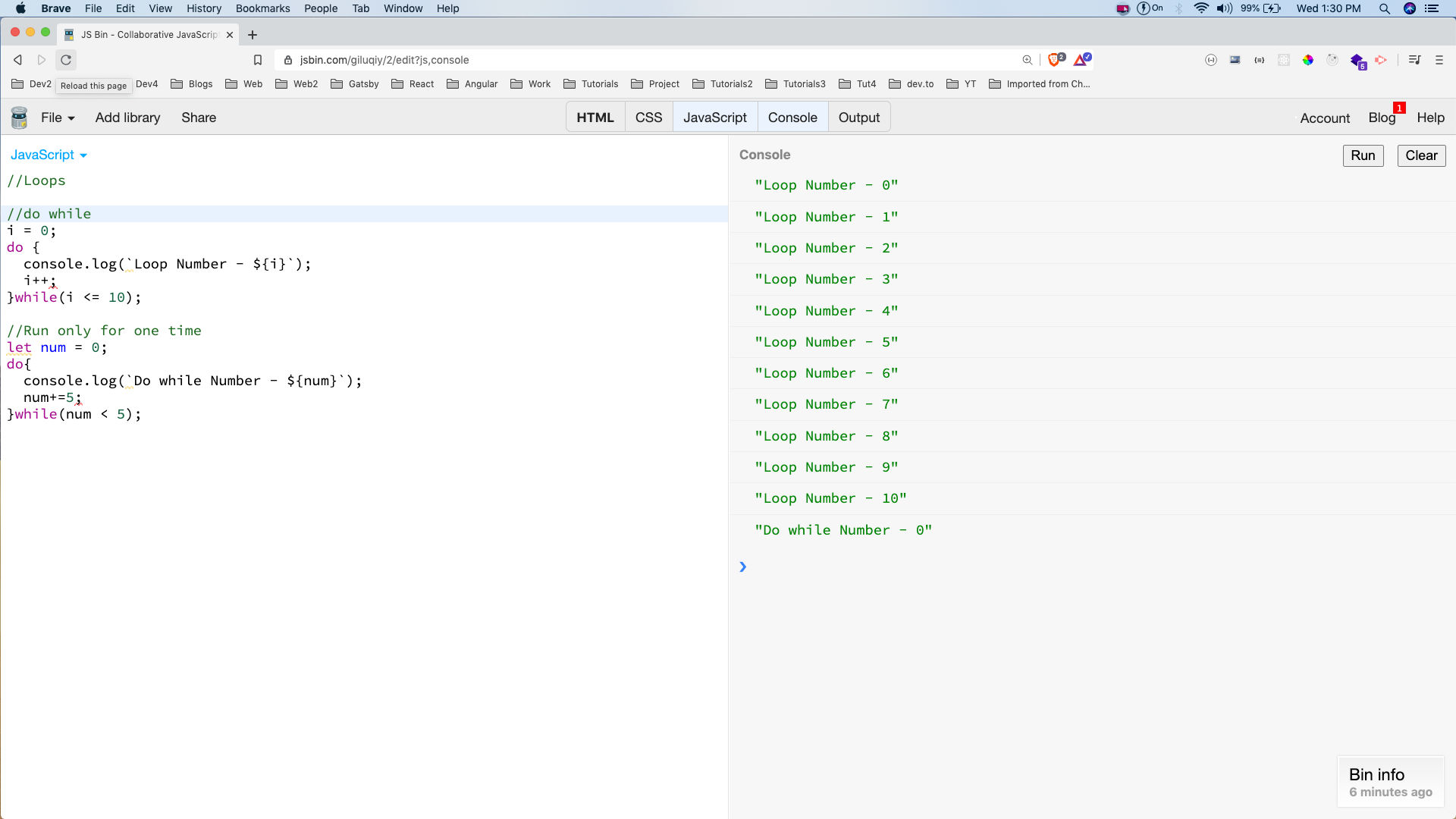do-while loop

One of the most important use of loops is to iterate over an array of object in JavaScript.

We have a simple example below where we have an array of objects lang, containing six languages.

We are iterating over it using the traditional for loop. Notice, that we are using lang.length which will return 6. So, the loop will run six times and inside it we are first taking the object with lang[i] and after that we can print any of it’s value and we are printing name here.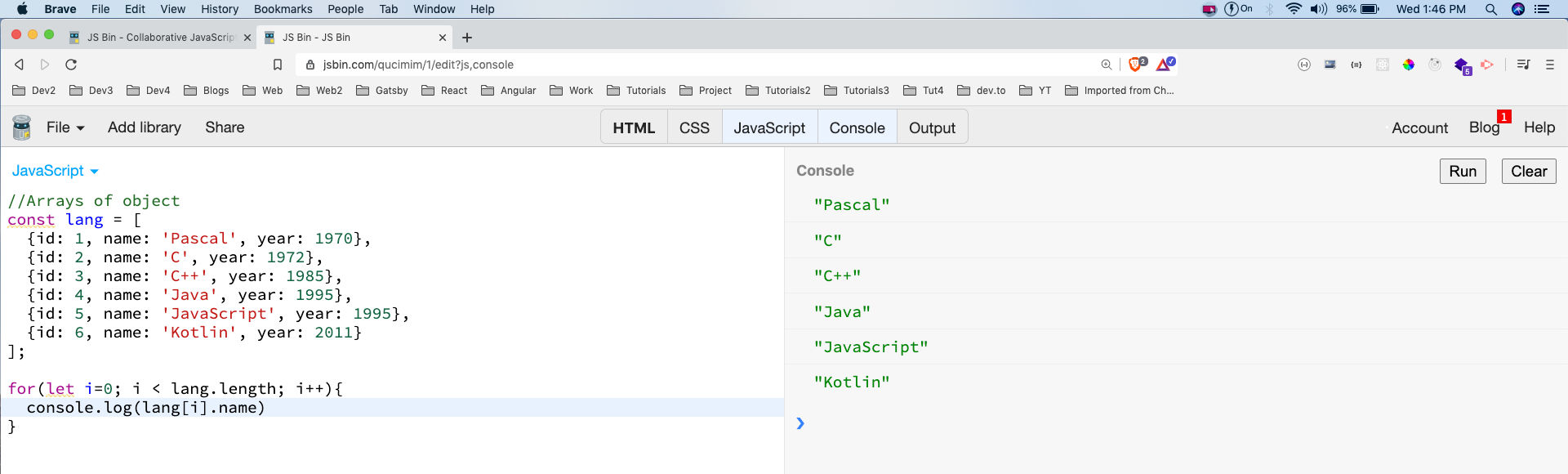Array of objects

Next, we will look into another form of for loop which is for..of loop. It have a much cleaner syntax and we don’t have to worry about incrementing the element or checking the condition, as it is all taken care by JavaScript.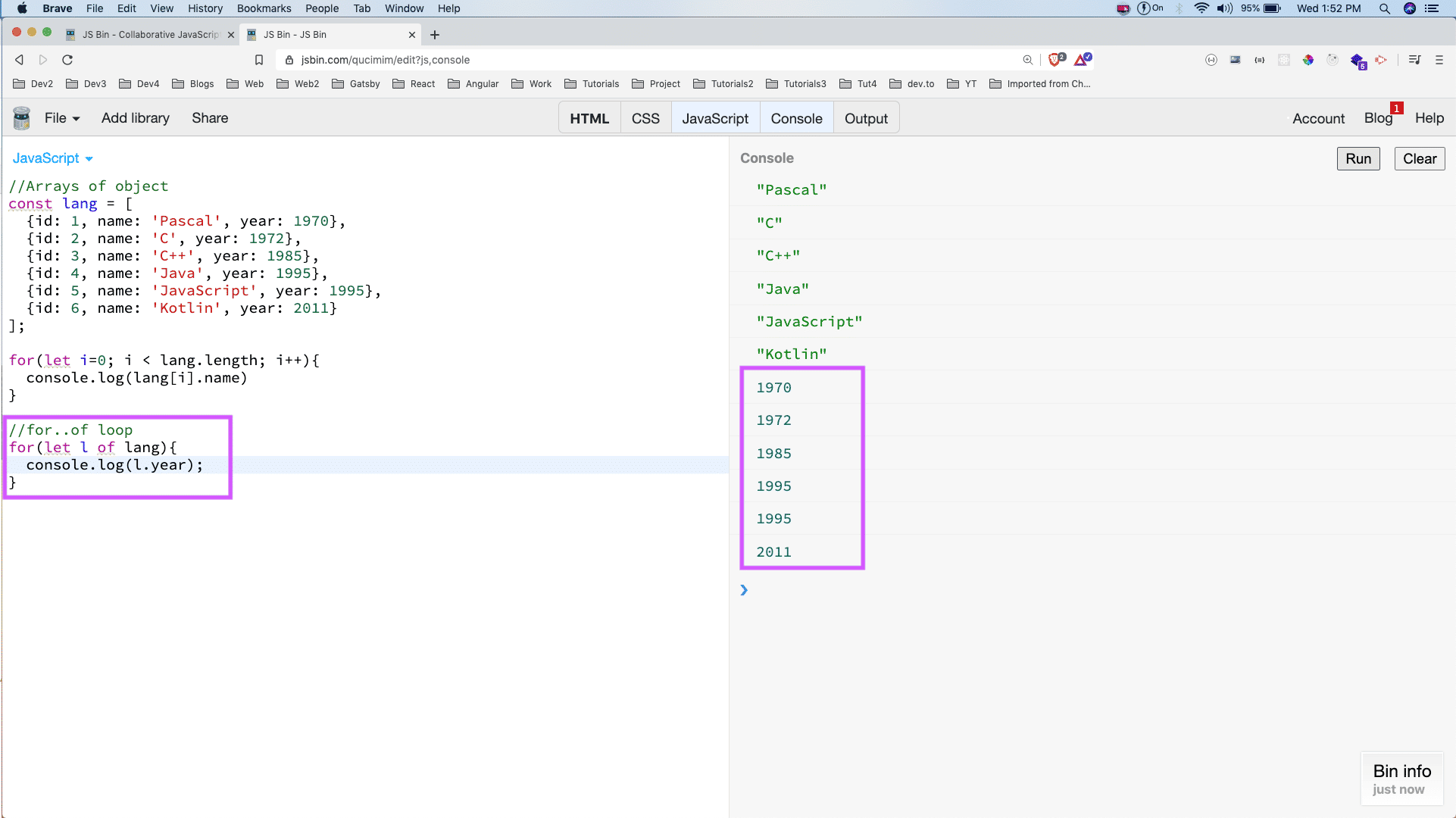for of loop

This completes part-3 of the series.

You can find the jsbins used in this part below.

https://jsbin.com/giluqiy/edit?js,console

https://jsbin.com/qucimim/edit?js,console